# Combinations

How many elements can form six times more combinations fourth class than combination of the second class?

Correct result:

n =  11

#### Solution:

Our quadratic equation calculator calculates it.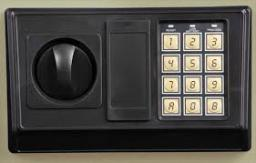We would be pleased if you find an error in the word problem, spelling mistakes, or inaccuracies and send it to us. Thank you!Tips to related online calculators
Looking for help with calculating roots of a quadratic equation?
Would you like to compute count of combinations?

## Next similar math problems:

• 2nd class combinationsFrom how many elements you can create 4560 combinations of the second class?
• CombinationsFrom how many elements we can create 990 combinations 2nd class without repeating?
• CombinationsIf the number of elements increase by 3, it increases the number of combinations of the second class of these elements 5 times. How many are the elements?
• Variations 4/2Determine the number of items when the count of variations of fourth class without repeating is 600 times larger than the count of variations of second class without repetition.
• Points in space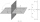There are n points, of which no three lie on one line and no four lies on one plane. How many planes can be guided by these points? How many planes are there if there are five times more than the given points?
• 2nd class variationsFrom how many elements you can create 2450 variations of the second class?
• First class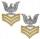The shipment contains 40 items. 36 are first grade, 4 are defective. How many ways can select 5 items, so that it is no more than one defective?
• Elements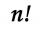If the number of elements is decreased by two the number of permutations is decreased 30 times. How many elements are?
• Variations 3rd classFrom how many elements we can create 13,800 variations 3rd class without repeating?
• VariationsDetermine the number of items when the count of variations of fourth class without repeating is 42 times larger than the count of variations of third class without repetition.
• Cinema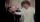How many ways can be divided 11 free tickets to the premiere of "Jáchyme throw it in the machine" between 6 pensioners?
• Soccer teams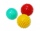Have to organize soccer teams. There are 3 age groups. How many different ways can you organize teams of ten for each age group? Is this a permutation or combination?
• ExaminationThe class is 21 students. How many ways can choose two to examination?
• Party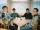At the party everyone clink with everyone. Together, they clink 406 times. How many people were at the party?
• Committees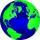How many different committees of 2 people can be formed from a class of 21 students?
• FruitsIn the shop sell 4 kinds of fruits. How many ways can we buy three pieces of fruit?
• Chocolates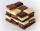In the market have 3 kinds of chocolates. How many ways can we buy 14 chocolates?